# RS Aggarwal Solutions for Class 8 Maths Chapter 17 - Construction of Quadrilaterals Exercise 17B

Students can refer and download RS Aggarwal Solutions for Class 8 Maths Chapter 17- Exercise 17B, Construction of Quadrilaterals from the links provided below. Our experts have solved the RS Aggarwal Solutions to help students excel in their exams. In Exercise 17B of RS Aggarwal Class 8 Maths, we shall brief all the topics covered in Chapter 17.

## Download PDF of RS Aggarwal Solutions for Class 8 Maths Chapter 17- Construction of Quadrilaterals Exercise 17B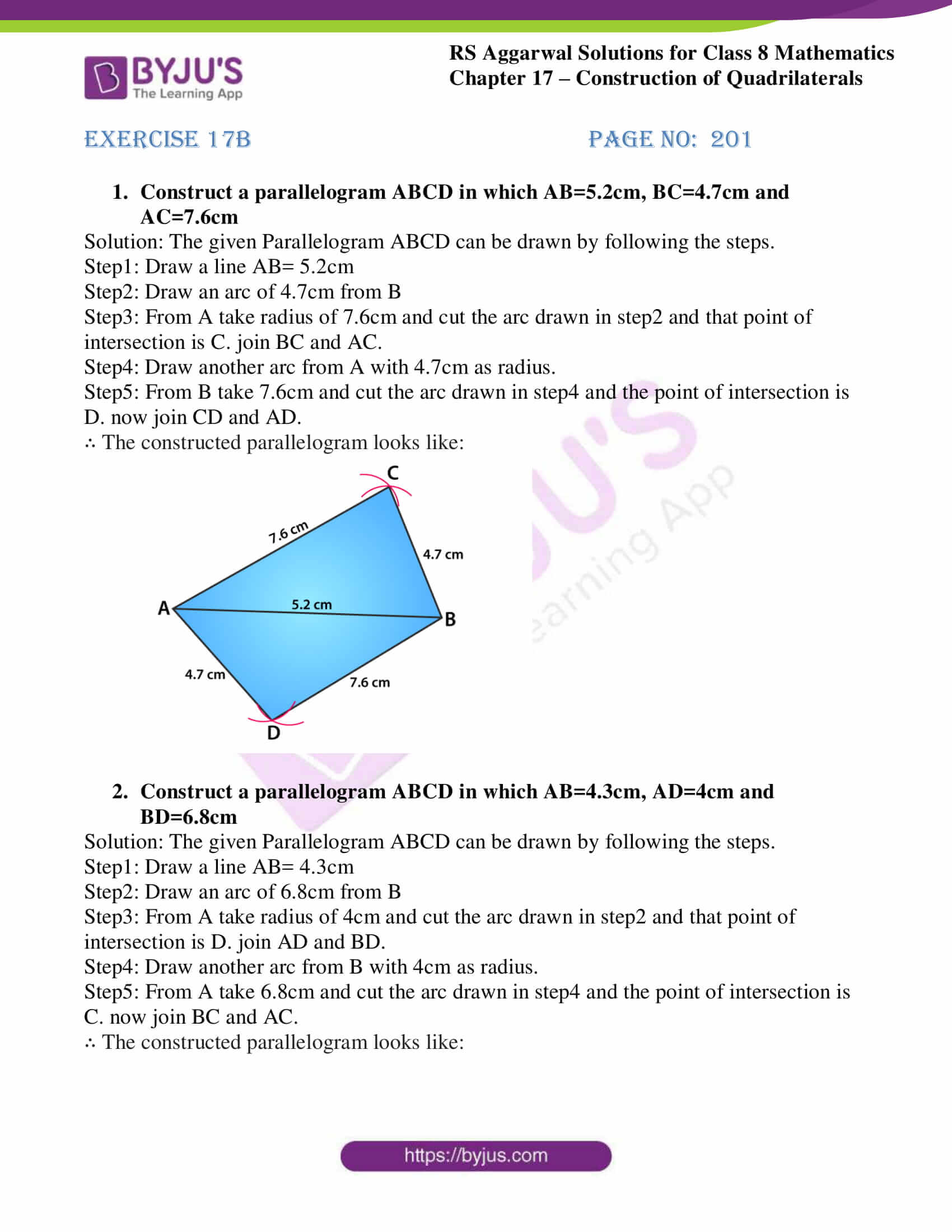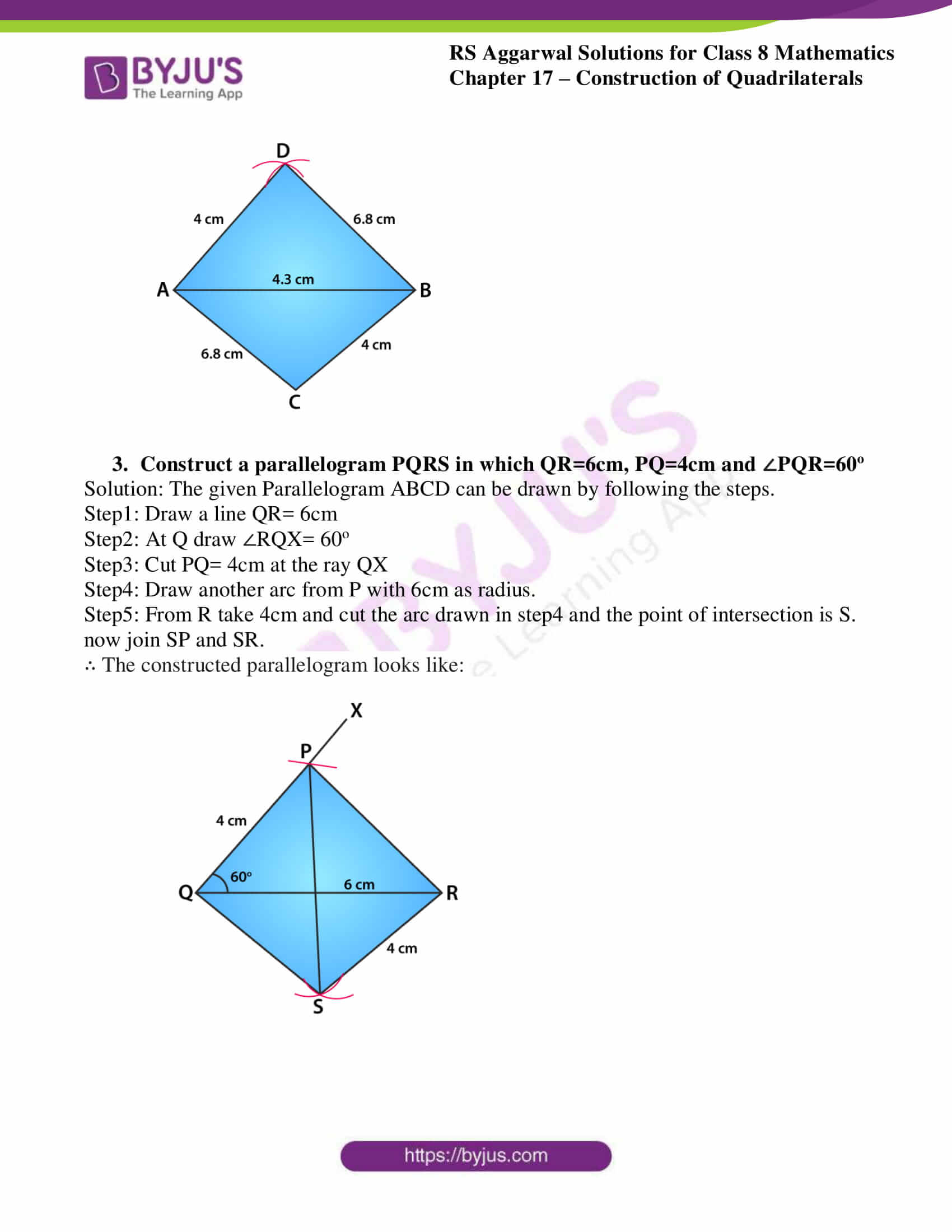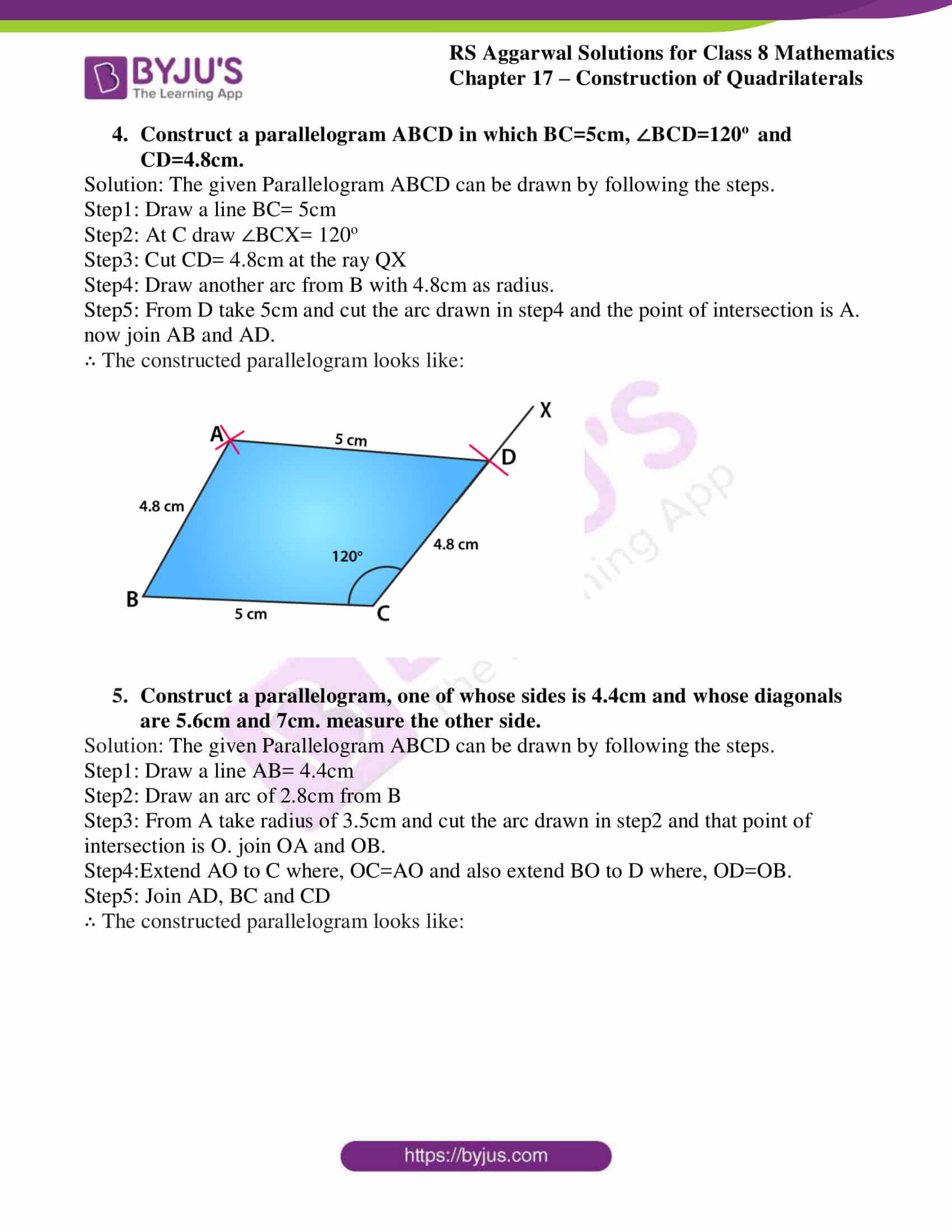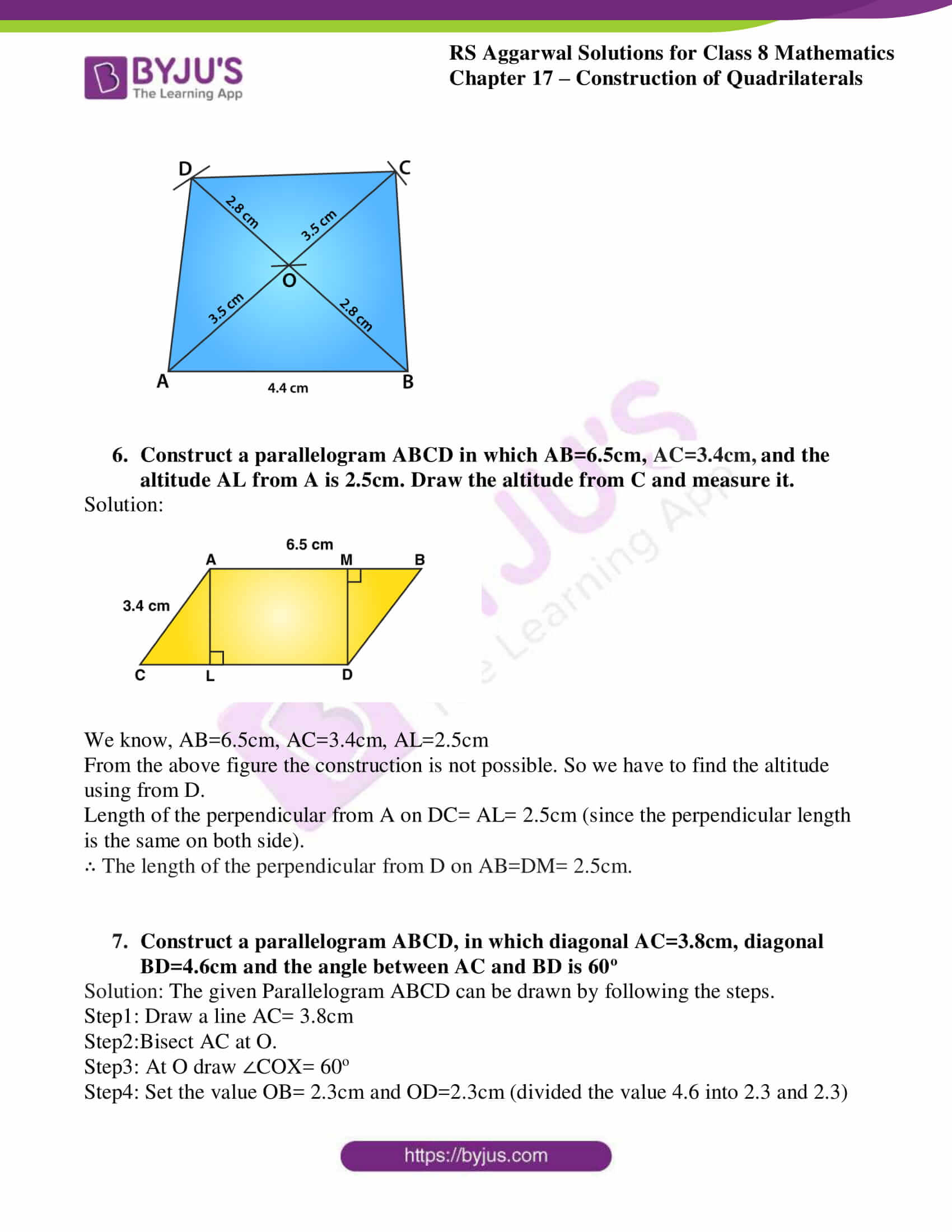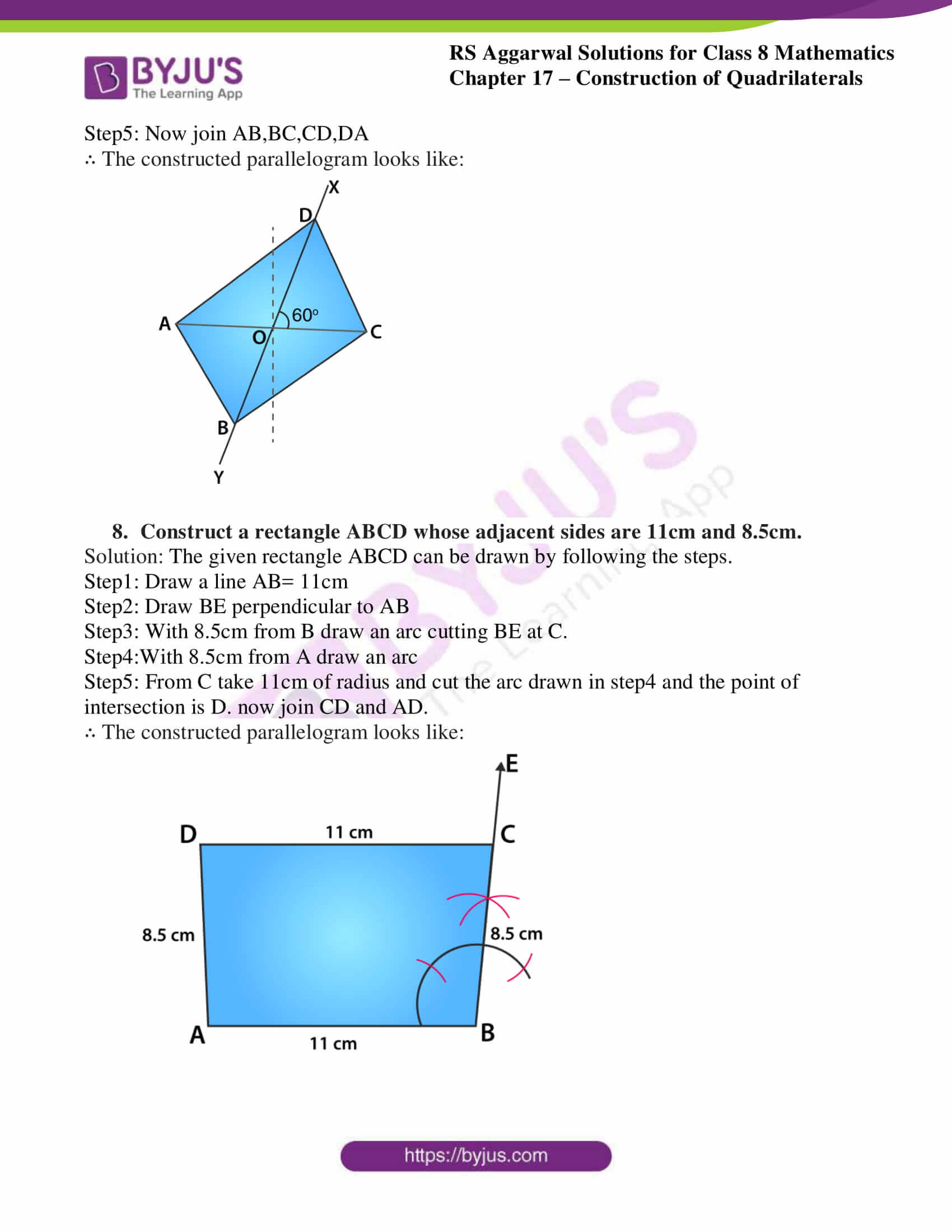### Access Answers to RS Aggarwal Solutions for Class 8 Maths Chapter 17- Construction of Quadrilaterals Exercise 17B

1. Construct a parallelogram ABCD in which AB=5.2cm, BC=4.7cm and AC=7.6cm

Solution: The given Parallelogram ABCD can be drawn by following the steps.

Step1: Draw a line AB= 5.2cm

Step2: Draw an arc of 4.7cm from B

Step3: From A take radius of 7.6cm and cut the arc drawn in step2 and that point of intersection is C. join BC and AC.

Step4: Draw another arc from A with 4.7cm as radius.

Step5: From B take 7.6cm and cut the arc drawn in step4 and the point of intersection is D. now join CD and AD.

∴ The constructed parallelogram looks like: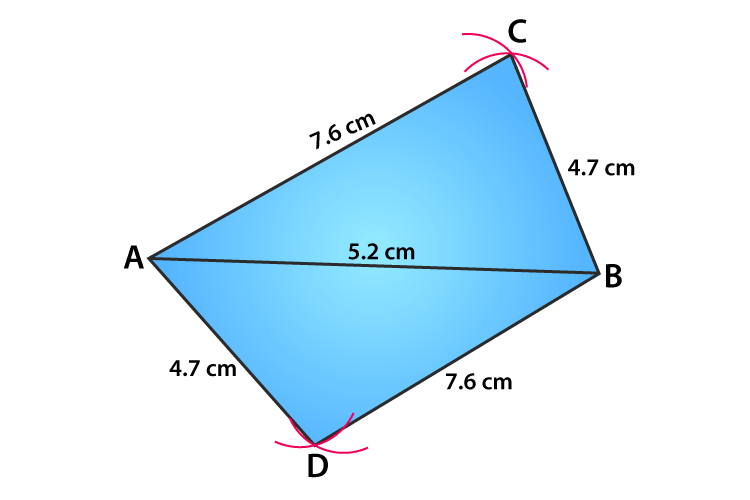2. Construct a parallelogram ABCD in which AB=4.3cm, AD=4cm and BD=6.8cm

Solution: The given Parallelogram ABCD can be drawn by following the steps.

Step1: Draw a line AB= 4.3cm

Step2: Draw an arc of 6.8cm from B

Step3: From A take radius of 4cm and cut the arc drawn in step2 and that point of intersection is D. join AD and BD.

Step4: Draw another arc from B with 4cm as radius.

Step5: From A take 6.8cm and cut the arc drawn in step4 and the point of intersection is C. now join BC and AC.

∴ The constructed parallelogram looks like: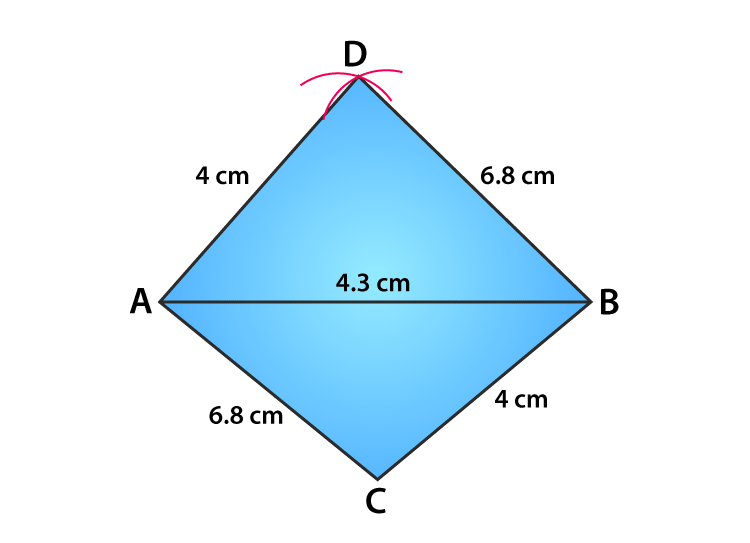3. Construct a parallelogram PQRS in which QR=6cm, PQ=4cm and ∠PQR=60o

Solution: The given Parallelogram ABCD can be drawn by following the steps.

Step1: Draw a line QR= 6cm

Step2: At Q draw ∠RQX= 60o

Step3: Cut PQ= 4cm at the ray QX

Step4: Draw another arc from P with 6cm as radius.

Step5: From R take 4cm and cut the arc drawn in step4 and the point of intersection is S. now join SP and SR.

∴ The constructed parallelogram looks like: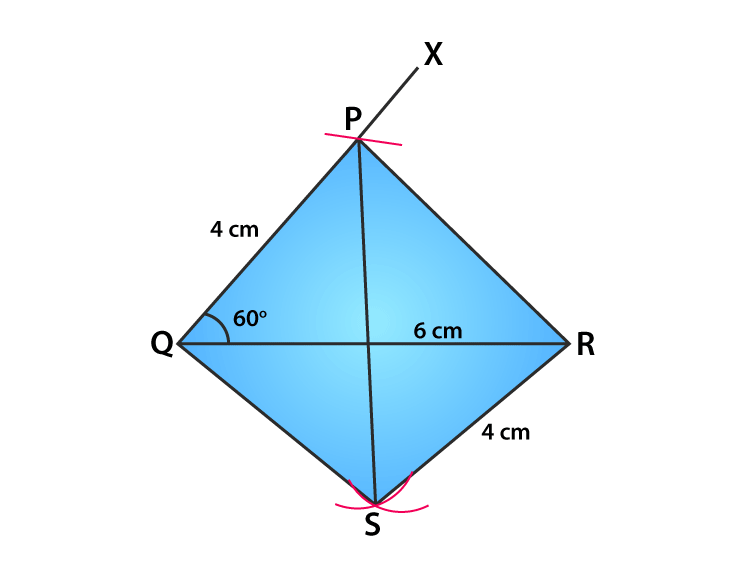4. Construct a parallelogram ABCD in which BC=5cm, ∠BCD=120o and CD=4.8cm.

Solution: The given Parallelogram ABCD can be drawn by following the steps.

Step1: Draw a line BC= 5cm

Step2: At C draw ∠BCX= 120o

Step3: Cut CD= 4.8cm at the ray QX

Step4: Draw another arc from B with 4.8cm as radius.

Step5: From D take 5cm and cut the arc drawn in step4 and the point of intersection is A. now join AB and AD.

∴ The constructed parallelogram looks like: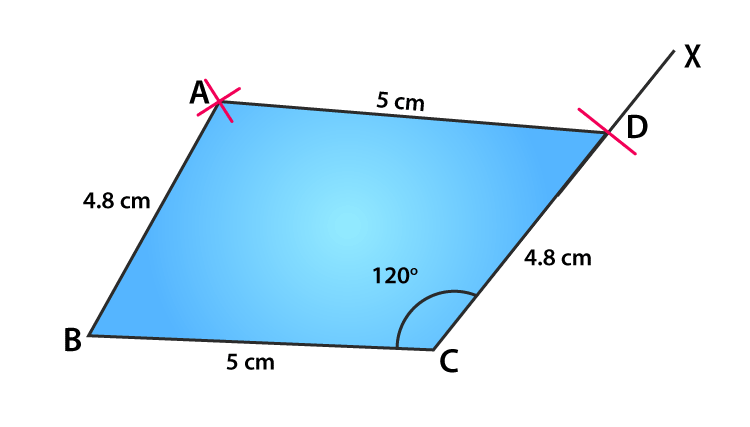5. Construct a parallelogram, one of whose sides is 4.4cm and whose diagonals are 5.6cm and 7cm. measure the other side.

Solution: The given Parallelogram ABCD can be drawn by following the steps.

Step1: Draw a line AB= 4.4cm

Step2: Draw an arc of 2.8cm from B

Step3: From A take radius of 3.5cm and cut the arc drawn in step2 and that point of intersection is O. join OA and OB.

Step4:Extend AO to C where, OC=AO and also extend BO to D where, OD=OB.

Step5: Join AD, BC and CD

∴ The constructed parallelogram looks like: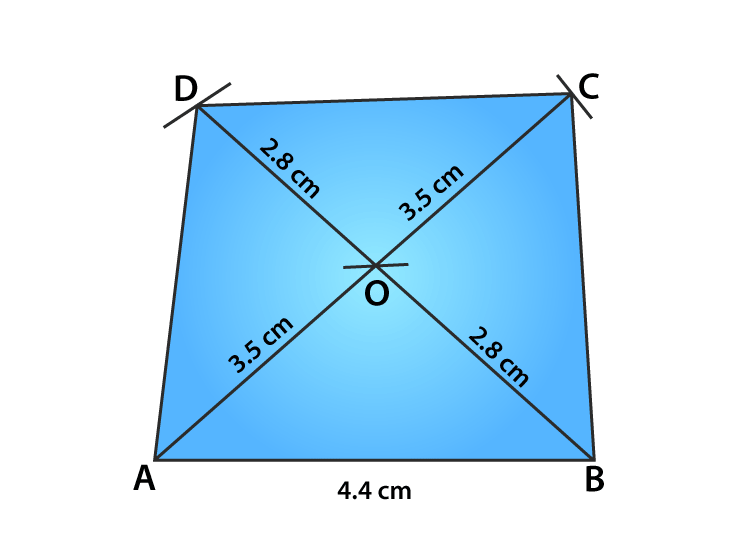6. Construct a parallelogram ABCD in which AB=6.5cm, AC=3.4cm, and the altitude AL from A is 2.5cm. Draw the altitude from C and measure it.

Solution: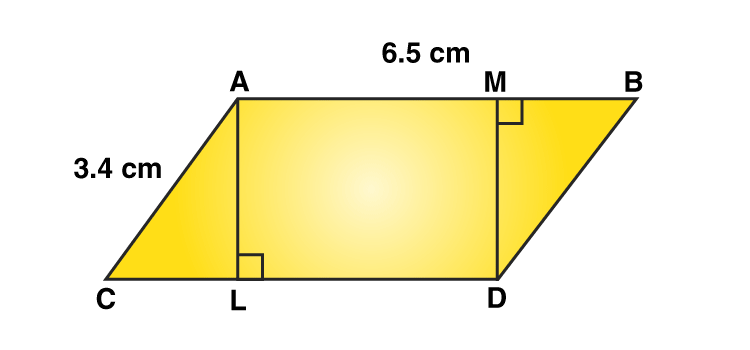We know, AB=6.5cm, AC=3.4cm, AL=2.5cm

From the above figure the construction is not possible. So we have to find the altitude using from D.

Length of the perpendicular from A on DC= AL= 2.5cm (since the perpendicular length is the same on both side).

∴ The length of the perpendicular from D on AB=DM= 2.5cm.

7. Construct a parallelogram ABCD, in which diagonal AC=3.8cm, diagonal BD=4.6cm and the angle between AC and BD is 60o

Solution: The given Parallelogram ABCD can be drawn by following the steps.

Step1: Draw a line AC= 3.8cm

Step2:Bisect AC at O.

Step3: At O draw ∠COX= 60o

Step4: Set the value OB= 2.3cm and OD=2.3cm (divided the value 4.6 into 2.3 and 2.3)

Step5: Now join AB,BC,CD,DA

∴ The constructed parallelogram looks like: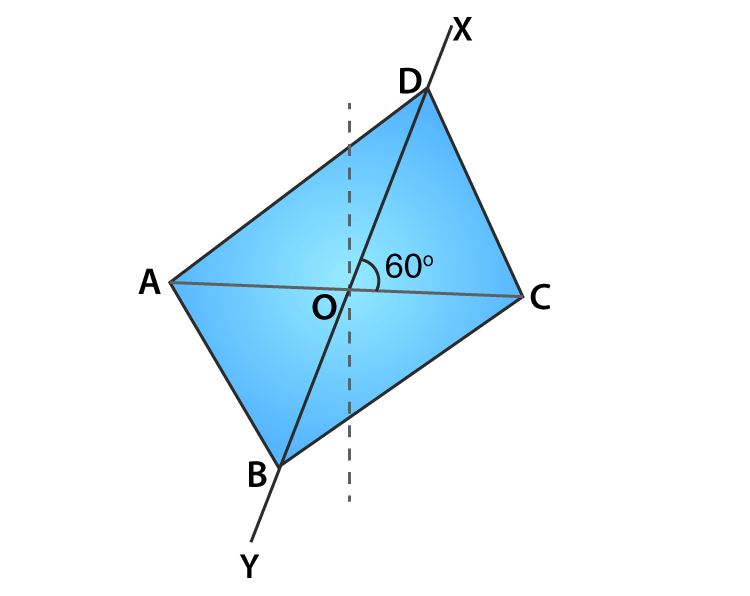8. Construct a rectangle ABCD whose adjacent sides are 11cm and 8.5cm.

Solution: The given rectangle ABCD can be drawn by following the steps.

Step1: Draw a line AB= 11cm

Step2: Draw BE perpendicular to AB

Step3: With 8.5cm from B draw an arc cutting BE at C.

Step4:With 8.5cm from A draw an arc

Step5: From C take 11cm of radius and cut the arc drawn in step4 and the point of intersection is D. now join CD and AD.

∴ The constructed parallelogram looks like: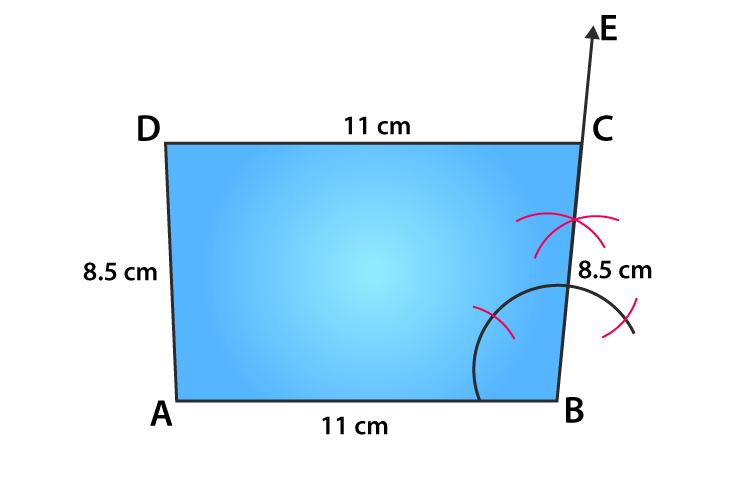### Access other Exercise of RS Aggarwal Solutions for Class 8 Maths Chapter 17- Construction of Quadrilaterals

Exercise 17A Solutions 13 Questions

## RS Aggarwal Solutions for Class 8 Maths Chapter 17- Construction of Quadrilaterals Exercise 17B

Exercise 17B of RS Aggarwal Class 8, Construction of Quadrilaterals. This exercise mainly consists of Objective Questions related to the topics discussed earlier in Chapter 17.

Students are suggested to try solving the questions from RS Aggarwal book of Class 8 and then refer to these solutions to know the best way of approaching the different questions. By referring to these solutions, students develop problem-solving abilities and can tackle any type of questions easily. Thus practicing as many times as possible helps in building time management skills and also boosts the confidence level to achieve high marks.

#### 1 Comment

1. Rudranil Saha

Very good